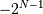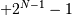# numpy.invert¶

`numpy.``invert`(x, /, out=None, *, where=True, casting='same_kind', order='K', dtype=None, subok=True[, signature, extobj]) = <ufunc 'invert'>

Compute bit-wise inversion, or bit-wise NOT, element-wise.

Computes the bit-wise NOT of the underlying binary representation of the integers in the input arrays. This ufunc implements the C/Python operator `~`.

For signed integer inputs, the two’s complement is returned. In a two’s-complement system negative numbers are represented by the two’s complement of the absolute value. This is the most common method of representing signed integers on computers [R35]. A N-bit two’s-complement system can represent every integer in the rangeto.

Parameters: x : array_like Only integer and boolean types are handled. out : ndarray, None, or tuple of ndarray and None, optional A location into which the result is stored. If provided, it must have a shape that the inputs broadcast to. If not provided or None, a freshly-allocated array is returned. A tuple (possible only as a keyword argument) must have length equal to the number of outputs. where : array_like, optional Values of True indicate to calculate the ufunc at that position, values of False indicate to leave the value in the output alone. **kwargs For other keyword-only arguments, see the ufunc docs. out : array_like Result.

`binary_repr`
Return the binary representation of the input number as a string.

Notes

`bitwise_not` is an alias for `invert`:

```>>> np.bitwise_not is np.invert
True
```

References

 [R35] (1, 2) Wikipedia, “Two’s complement”, http://en.wikipedia.org/wiki/Two’s_complement

Examples

We’ve seen that 13 is represented by `00001101`. The invert or bit-wise NOT of 13 is then:

```>>> np.invert(np.array(, dtype=uint8))
array(, dtype=uint8)
>>> np.binary_repr(x, width=8)
'00001101'
>>> np.binary_repr(242, width=8)
'11110010'
```

The result depends on the bit-width:

```>>> np.invert(np.array(, dtype=uint16))
array(, dtype=uint16)
>>> np.binary_repr(x, width=16)
'0000000000001101'
>>> np.binary_repr(65522, width=16)
'1111111111110010'
```

When using signed integer types the result is the two’s complement of the result for the unsigned type:

```>>> np.invert(np.array(, dtype=int8))
array([-14], dtype=int8)
>>> np.binary_repr(-14, width=8)
'11110010'
```

Booleans are accepted as well:

```>>> np.invert(array([True, False]))
array([False,  True], dtype=bool)
```

#### Previous topic

numpy.bitwise_xor

numpy.left_shift